括号匹配 数据结构栈_数据结构实验之栈与队列四:括号匹配 测试数据 - CSDN
• 的应用括号匹配问题：原理：代码实现： 括号匹配问题： 原理： 1、括号匹配成功的情况：为空 2、括号匹配失败的情况： 　a、下一个为右括号但是和栈顶左括号不匹配 　ｂ、下一个是右括号但以空 　ｃ、所有的...
栈的应用括号匹配问题：原理：代码实现：
括号匹配问题：
原理：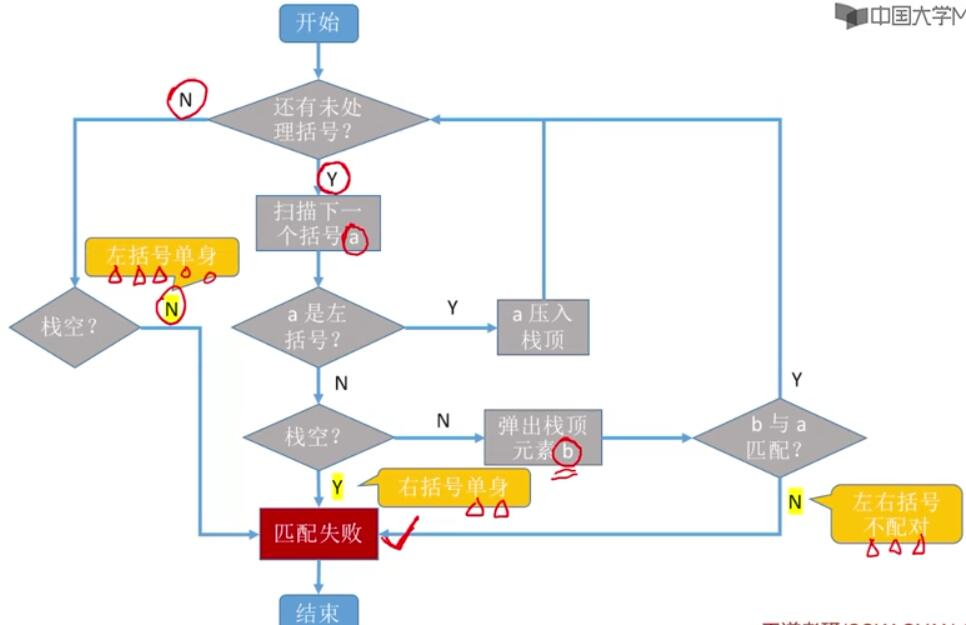1、括号匹配成功的情况：栈为空
2、括号匹配失败的情况：
a、下一个为右括号但是和栈顶左括号不匹配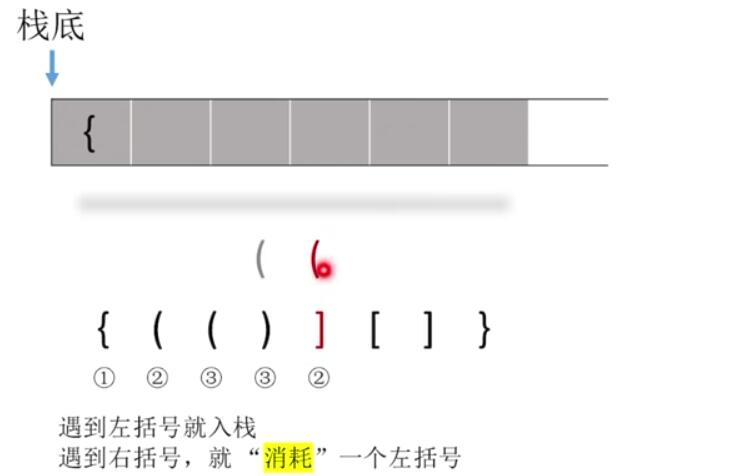ｂ、下一个是右括号但栈以空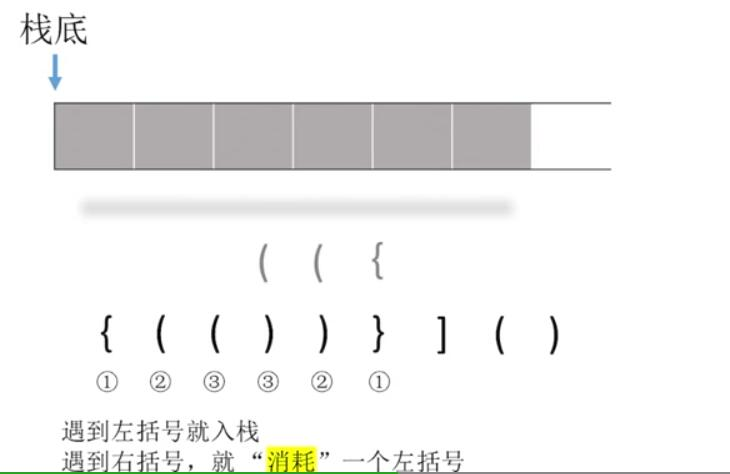ｃ、所有的空号都匹配完成后栈不空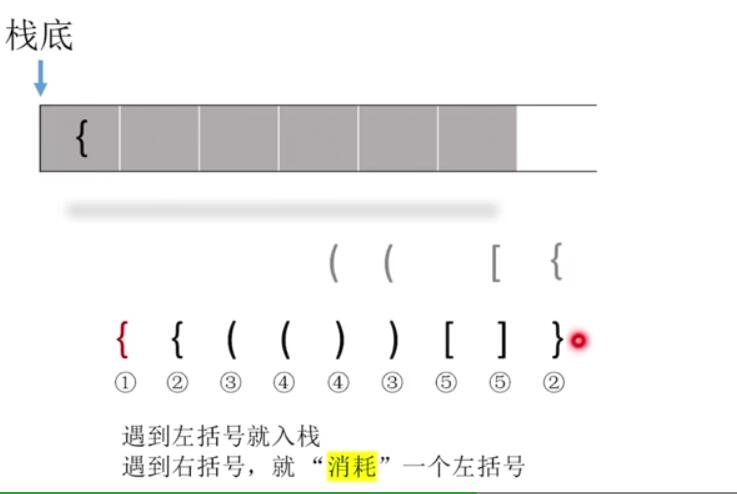代码实现：
1、方法一
bool isValid(char * s){
char *stack = (char*)malloc(strlen(s)+1);
int top = 0;
int i;
// if(s == ')' || s == ']' || s == '}')
//  return false;
for(i = 0;i < strlen(s);i ++){
if(s[i] == '(' || s[i] == '[' || s[i] == '{'){
top ++;
stack[top] = s[i];
}
else {
if((s[i] == ')' && stack[top] == '(') || (s[i] == ']' && stack[top] == '[') || (s[i] == '}' && stack[top] == '{'))
top --;
else
return false;
}
}
if(top == 0)
return true;
else
return false;
}

方法二：
bool isValid(char * s){
char *stack = (char*)malloc(strlen(s)+1);
int top = 0;
int i;
// if(s == ')' || s == ']' || s == '}')
//  return false;
for(i = 0;i < strlen(s);i ++){
if(s[i] == '(' || s[i] == '[' || s[i] == '{'){
top ++;
stack[top] = s[i];
}
else
//由ASCALL表可知
if((stack[top]+1) == s[i] || (stack[top]+2) == s[i])
top --;
else
return false;
}
}
if(top == 0)
return true;
else
return false;
}

方法三：
bool isValid(char *s){
//SqStack S;
char *Stack = (char*)malloc(strlen(s));
//InitStack(S);
int top = -1;
for(int i = 0;i < strlen(s);i ++){
if(s[i] == '(' || s[i] == '[' || s[i] == '{'){
top ++;
Stack[top] = s[i];
}
else{
if(top == -1)
return false;
char topElem;
if(s[i] == ')' && Stack[top] != '(')
return false;
if(s[i] == ']' && Stack[top] != '[')
return false;
if(s[i] == '}' && Stack[top] != '{')
return false;
top --;
}
}
if(top == -1)
return true;
else
return false;
}



展开全文• 括号匹配算法在各种编程的IDE工具中都会用到，用来检测关于括号匹配的语法错误，括号匹配实际上不复杂，主要就是利用这个数据结构，扫描输入的字符串，若遇到左括号则直接入栈，若遇到右括号则弹出栈顶括号，看...
括号匹配算法在各种编程的IDE工具中都会用到，用来检测关于括号匹配的语法错误，括号匹配实际上不复杂，主要就是利用栈这个数据结构，扫描输入的字符串，若遇到左括号则直接入栈，若遇到右括号则弹出栈顶括号，看是否与当前括号类型相同（如同为小括号()，或同为[]，注意括号应该是在英文输入法的情况下输入的），若相同则二者匹配，否则不匹配，另外，如果扫描完成，而栈中仍存在未匹配的括号，则说明不匹配，当且仅当扫描序列与栈中元素同时为空时才表示完全匹配。本博客将详细讲解括号匹配算法，为了将焦点聚焦在算法本身上，仅仅只对小括号进行检测是否匹配。
注：关于栈的另外一个典型应用就是表达式求值算法，类似于计算器效果，具体可以参看我的博客：算术表达式

# include<stdio.h>
# include<string.h>
# include<stdlib.h>
typedef struct node
{
char a;
struct node *pnext;
{
(s)->pnext=NULL;
}
{
(ptemp)->a=c;
ptemp->pnext=(top)->pnext;
(top)->pnext=ptemp;

}
{
ptemp=(top)->pnext;
*c=(ptemp)->a;
if(ptemp==NULL)
printf("栈空！");
(top)->pnext=ptemp->pnext;
free(ptemp);
}
{
*x=top->pnext->a;
}
{
if(s->pnext==NULL)
return 1;
else return 0;
}
int match(char x,char y)
{
if((x=='(')&&(y==')'))
return 1;
else return 0;
}
void Match(char x[])
{
node s;
int i;
char ch;
inistack(&s);
for(i=0;x[i]!='\0';i++)
{
if(x[i]=='(')
{
push(&s,x[i]);
}
else
if(isempty(&s))
{
printf("右括号多余!\n");
return;
}
else
{
get(&s,&ch);
if(match(ch,x[i]))
{
pop(&s,&ch);
}

else
{
printf("对应的括号不匹配!\n");
return;
}
}
}
if(isempty(&s))
printf("括号匹配!\n");
else
printf("左括号多余!\n");
}
void main()
{
int i;
char x;
printf("请输入只含小括号的字符串\n");
scanf("%s",x);
Match(x);
}
程序运行结果如下：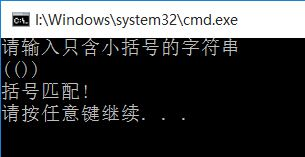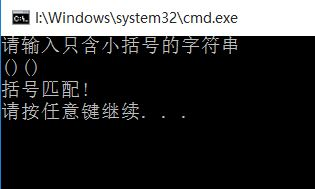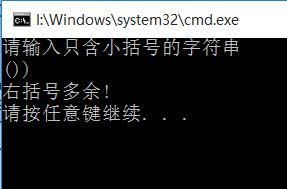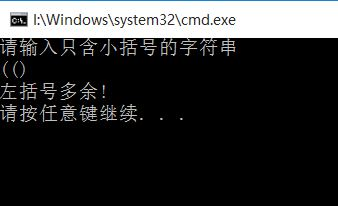展开全文数据结构 括号匹配
• 数据结构之---C语言实现括号匹配实现）
#include <stdio.h>
#include <stdlib.h>
#define STACKINCREAMENT 10
#define STACK_INIT_SIZE 100
#define OVERFLOW -2
#define OK 1
#define ERROR 0
typedef int status;
typedef char SElemtype;
typedef struct
{
SElemtype *base;
SElemtype *top;
status stacksize;
}sqstack;

//初始化栈
status Init(sqstack *s)
{
s->base = (SElemtype *)malloc(STACK_INIT_SIZE * sizeof(SElemtype));
if(!s->base)
exit(OVERFLOW);
s->top = s->base;
s->stacksize = STACK_INIT_SIZE ;
return OK;
}

//获取栈顶元素
status Gettop(sqstack *s, SElemtype e)
{
if(s->top == s->base)
return ERROR;
e = *(s->top-1);
return OK;
}

//压栈
status push(sqstack *s, SElemtype e)
{
if(s->top - s->base >= s->stacksize)
{
s->base = (SElemtype *)realloc(s->base, (s->stacksize + STACKINCREAMENT) * sizeof(SElemtype));
if(!s->base)
exit(OVERFLOW);
s->top = s->base + s->stacksize;
s->stacksize += STACKINCREAMENT;
}
*s->top++ = e;
return OK;
}

//出栈
status pop(sqstack *s, SElemtype *e)
{
if(s->top == s->base)
return ERROR;
*e=*--s->top;
return OK;
}

//判断是否为空栈
status stackempty(sqstack *s)
{
if(s->top==s->base)
return OK;
return ERROR;
}

//清空栈
status clearstack(sqstack *s)
{
if(s->top == s->base)
return ERROR;
s->top = s->base;
return OK;
}

//括号匹配算法
status Parenthesis_match(sqstack *s,char *str)
{
int i=0, flag=0;
SElemtype e;
while(str[i] != '\0')
{
switch(str[i])
{
case '(':
push(s,str[i]);
break;
case '[':
push(s,str[i]);
break;
case ')':
{
pop(s,&e);
if(e != '(')
flag=1;
}
break;
case ']':
{
pop(s,&e);
if(e!='[')
flag=1;
}
break;
default:
break;
}
if(flag)
break;
i++;
}

if(!flag && stackempty(s))
printf("括号匹配成功!\n");
else
printf("括号匹配失败!\n");
return OK;
}

int main()
{
char str, enter;
sqstack s;
Init(&s);
printf("请输入字符串:\n");
scanf("%s",str);
scanf("%c",&enter);
Parenthesis_match(&s,str);
return 0;
}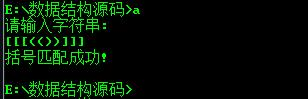展开全文• 问题描述：对一个字符串的左右括号进行匹配。 输入：一个字符串表达式 输出：匹配成功true或匹配失败false 例如：假设一个算术表达式中可以包含三种括号：圆括号“()”，方括号“[]”和花括号“{}”，且这三种...
问题描述：对一个字符串的左右括号进行匹配。

输入：一个字符串表达式

输出：匹配成功true或匹配失败false

例如：假设一个算术表达式中可以包含三种括号：圆括号“()”，方括号“[]”和花括号“{}”，且这三种括号可按任意的次序嵌套使用如：(a+b)[c*({d-e)]}，返回false。编写判别给定表达式中所含括号是否正确配对出现的算法。

匹配思想：通过观察可以发现，如果从左向右扫描一个字符串表达式，那么每个右括号都与最近扫描的那个未匹配的左括号相配。

算法思想：

因此我们可以在从左至右的扫描过程中把所有遇到的左括号存放到栈中，

每当遇到一个右括号的时候，检查栈是否为空，如果为空则意味着多出一个右括号，返回false；而如果栈不为空，就将它与栈顶的左括号相匹配：如果括号类型相同，就认为匹配成功继续向下读入，并从栈顶删除掉该左括号；否则返回false。

另外,在算法的开始和结束时,栈都应该是空的.所以匹配到最后还要判断栈是否为空,若非空，则说明多了左括号，匹配失败。

public class BracketMatch9
{

public boolean isMatch(String s)
{
Stack<Character> stack = new Stack<>();

//从左至右扫描字符串
for(int i =0;i<s.length();i++)
{
char temp = s.charAt(i);
switch(s.charAt(i))//switch 语句用于基于不同的条件来执行不同的动作。
{
//如果读入左括号则入栈
case '(':
case '[':
case '{':

stack.push(temp);//java5.0后引入自动装箱，char会自动转化为Character对象
break;//继续向下读入

//如果读入右括号，则看是否与栈顶左括号匹配
case ')':
case ']':
case '}':

if(stack.empty())//栈为空，说明多出右括号
return false;
else
{
//如果读入的右括号与栈顶的左括号类型相同，则让该左括号出栈，然后继续向下扫描
if((temp==')'&&stack.peek()=='(')||(temp ==']'&&stack.peek()=='[')||(temp=='}'&&stack.peek()=='{'))

{
stack.pop();
}
}

break;
}

}

//扫描完字符串后，如果栈非空，则说明多了左括号没匹配
if(!stack.empty())
return false;

return true;
}

public static void main(String[] args) {

boolean isMatch= new BracketMatch9().isMatch("(a+b)[c*(d-e)]");
System.out.println(isMatch+"");

}
}


展开全文括号匹配
• 数据结构——实现括号匹配 真正学习之后，才发现那些所谓的大婶不过是多用功了些。不知道以前为什么不亲自动手做做，原来这么简单。 #include #include #include #include /**的链式存储**/ typedef ...数据结构 括号匹配
• 检验算法借助一个，每当读入一个左括号，则直接入栈，等待相匹配的同类右括号；每当读入一个右括号，若与当前栈顶的左括号类型相同，则二者匹配，将栈顶的左括号出栈，直到表达式扫描完毕在处理过程中，还要考虑...
• 同样是用模板写的，实现上比慕课网上讲的简单一些，没有定义两个，而是直接...最后看mystack中栈顶是否为0，为0则打印括号匹配。 代码如下，写的不好还请大家指出共同讨论 demo.cpp #include #include "My数据结构c c++ 括号匹配
• 括号匹配的检验: eg: [([][][)]] 不匹配 [([][])] 匹配思路： 0x0.首先建立两个，并对其初始化 0x1.对表达式进行遍历，将相邻两个不能匹配的入栈到A，然后检测空间A是否为偶数，如果是表明有存在的可能，...
• 相信大家对这个名词都是有一些了解的。但是具体什么是呢？的作用？我们先来看一下百度百科给的解释: （stack）又名堆栈，它是一种运算受限的线性表。其限制是仅允许在表的一端进行插入和删除运算。这一端被...数据结构
• 解决办法：用c++stl中提供的数据结构stack 分析：用一个字符串保存输入的表达式，去遍历字符串，将字符串中的‘（’ 、‘ [ ’、‘ {’ 三个字符压入栈中，当遇到右括号时取出栈顶元素与之比较，若不匹配，则整个...
• /************************ author's email:...date:2018.1.1 顺序及其应用（括号匹配） ************************/ #include <iostream> using namespace std; #define maxSize 50 typedef struct...数据结构 顺序栈 括号匹配
• 所谓括号校验匹配其实质是对多种类型括号正确配对的校验（包括：()、[]、{}）即([])或者[()]为正确的表达式，如果出现交叉则匹配失败，如[(])或([())则为不正确格式。 该程序也运用了的思想。若是左括号则入栈，...括号匹配
• 本文是针对数据结构基础系列网络课程(3)：和队列的实现项目。【项目3 - 括号的匹配】 假设表达式中允许三种括号：圆括号、方括号和大括号。编写一个算法，判断表达式中的各种左括号是否与右括号匹配。 例如，...数据结构 应用
• 给定一个字符串，其中的字符只包含三种括号：花括号{ }、中括号[ ]、圆...括号匹配要求括号必须以正确的顺序配对，如“{ [ ] ( ) }”或 “[ ( { } [ ] ) ]” 等为正确的格式，而“[ ( ] )”或“{ [ ( ) }”或“( { ...数据结构 括号匹配 算法
• 利用括号匹配算法 C语言数据结构 利用括号匹配算法 C语言数据结构
• 括号匹配： 核心代码： bool BracketsCheck(char str[]){ LinkList St; char *p=str; char e; InitStack(St); while(*p!='\0'){ //printf("%c\n",*p); if(*p=='{'||*p=='['||*p=='(')...
• 利用栈数据结构解决括号匹配问题 题目描述： 在文字处理软件或编译程序设计时，常常需要检查一个字符串或一个 表达式中的括号是否相匹配?利用数据结构的“”机制，设计算法并 编写程序，判断表达式中括号匹配问题...数据结构 算法
• 题目描述现在，有一行括号序列，里面只包含”(“,”)”,”[“,”]”四种符号，请你检查这行括号是否配对。 如： []是匹配的 ([])[]是匹配的 ((]是不匹配的 ([)]是不匹配的 求解思想这里提供了两种求解方法：一...C语言
• 括号必须用相同类型的右括号闭合。 左括号必须以正确的顺序闭合。 注意空字符串可被认为是有效字符串。 参考leetcode.com或leetcode-cn.com  第20题 有效的括号 地址：...有效的括号 stack
...